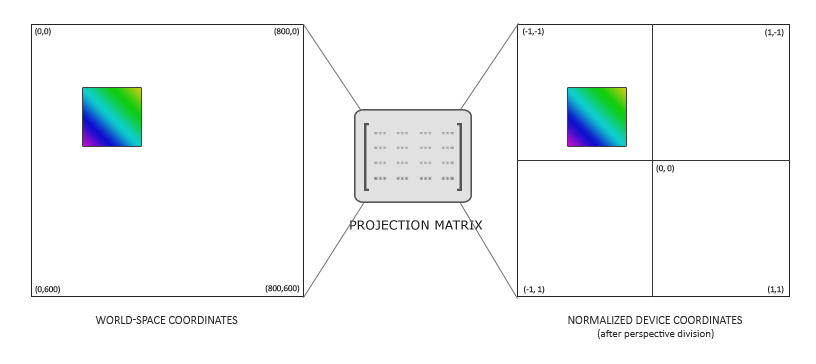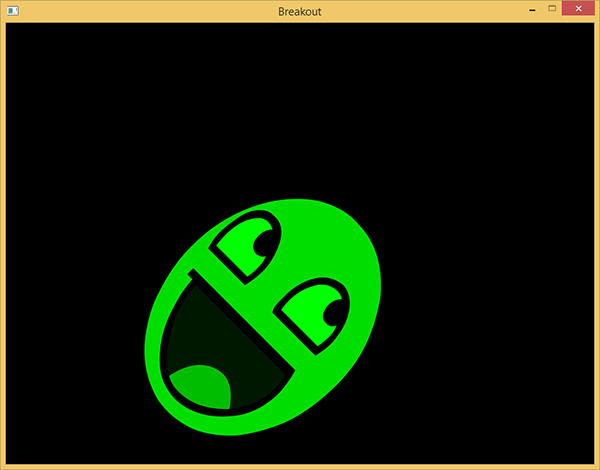# 渲染精灵

Note

## 2D投影矩阵

glm::mat4 projection = glm::ortho(0.0f, 800.0f, 600.0f, 0.0f, -1.0f, 1.0f);## 渲染精灵

Important

#version 330 core
layout (location = 0) in vec4 vertex; // <vec2 position, vec2 texCoords>

out vec2 TexCoords;

uniform mat4 model;
uniform mat4 projection;

void main()
{
TexCoords = vertex.zw;
gl_Position = projection * model * vec4(vertex.xy, 0.0, 1.0);
}


#version 330 core
in vec2 TexCoords;
out vec4 color;

uniform sampler2D image;
uniform vec3 spriteColor;

void main()
{
color = vec4(spriteColor, 1.0) * texture(image, TexCoords);
}


class SpriteRenderer
{
public:
~SpriteRenderer();

void DrawSprite(Texture2D &texture, glm::vec2 position,
glm::vec2 size = glm::vec2(10, 10), GLfloat rotate = 0.0f,
glm::vec3 color = glm::vec3(1.0f));
private:

void initRenderData();
};


SpriteRenderer类封装了一个着色器对象，一个顶点数组对象以及一个渲染和初始化函数。它的构造函数接受一个着色器对象用于之后的渲染。

### 初始化

void SpriteRenderer::initRenderData()
{
// 配置 VAO/VBO
GLuint VBO;
GLfloat vertices[] = {
// 位置     // 纹理
0.0f, 1.0f, 0.0f, 1.0f,
1.0f, 0.0f, 1.0f, 0.0f,
0.0f, 0.0f, 0.0f, 0.0f,

0.0f, 1.0f, 0.0f, 1.0f,
1.0f, 1.0f, 1.0f, 1.0f,
1.0f, 0.0f, 1.0f, 0.0f
};

glGenBuffers(1, &VBO);

glBindBuffer(GL_ARRAY_BUFFER, VBO);
glBufferData(GL_ARRAY_BUFFER, sizeof(vertices), vertices, GL_STATIC_DRAW);

glEnableVertexAttribArray(0);
glVertexAttribPointer(0, 4, GL_FLOAT, GL_FALSE, 4 * sizeof(GLfloat), (GLvoid*)0);
glBindBuffer(GL_ARRAY_BUFFER, 0);
glBindVertexArray(0);
}


### 渲染


void SpriteRenderer::DrawSprite(Texture2D &texture, glm::vec2 position,
glm::vec2 size, GLfloat rotate, glm::vec3 color)
{
// 准备变换
glm::mat4 model;
model = glm::translate(model, glm::vec3(position, 0.0f));

model = glm::translate(model, glm::vec3(0.5f * size.x, 0.5f * size.y, 0.0f));
model = glm::rotate(model, rotate, glm::vec3(0.0f, 0.0f, 1.0f));
model = glm::translate(model, glm::vec3(-0.5f * size.x, -0.5f * size.y, 0.0f));

model = glm::scale(model, glm::vec3(size, 1.0f));

glActiveTexture(GL_TEXTURE0);
texture.Bind();

glDrawArrays(GL_TRIANGLES, 0, 6);
glBindVertexArray(0);
}## 你好，精灵

SpriteRenderer  *Renderer;

void Game::Init()
{
// 加载着色器
// 配置着色器
glm::mat4 projection = glm::ortho(0.0f, static_cast<GLfloat>(this->Width),
static_cast<GLfloat>(this->Height), 0.0f, -1.0f, 1.0f);
// 设置专用于渲染的控制
// 加载纹理
}


void Game::Render()
{
Renderer->DrawSprite(ResourceManager::GetTexture("face"),
glm::vec2(200, 200), glm::vec2(300, 400), 45.0f, glm::vec3(0.0f, 1.0f, 0.0f));
}